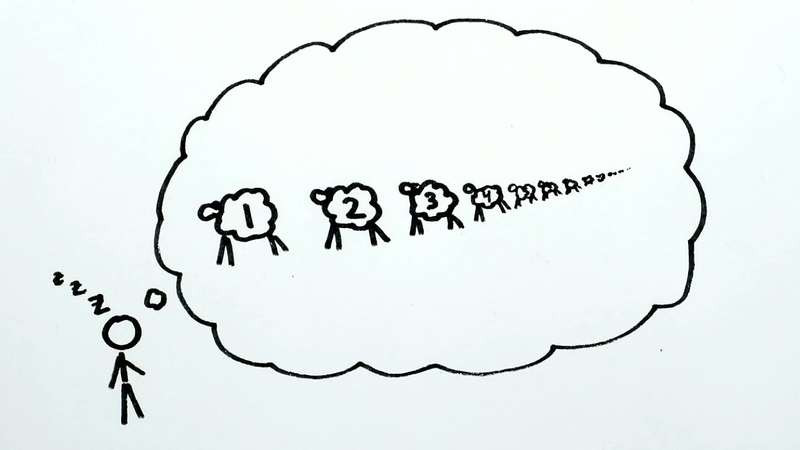Search

# To Infinity ... and Beyond!!

From calculus to infinite series, explore the mind-boggling properties of infinity and the role it plays in the world of mathematics.Don't worry, if you didn't cringe at the title or the sub-heading, I definitely did. Mathematics can be a rather dry and boring subject to write about in blogs but infinity is one of the few topics that can really twist your mind and poke that little curiosity bubble in your head.

We all have heard of infinity in many different contexts: infinite money, infinite wishes, infinite lives, infinity gems.... the list goes on. What about in mathematics?

Well, surprisingly, there isn't a fixed definition of infinity up to this very day due to the complexity surrounding it. At best, infinity is :

The concept of something that is unlimited, endless, without bound.

Now fear not, we aren't going to delve into the conceptual and complicated parts of infinity. Let's start off by saying that we treat infinity as an immeasurably large value or a sort of point on the number line so far off into the distance that it can never be reached.

### Sizes of the infinite

Using this analogy, can we say that there are different sizes of infinity? Think of sets. A set is a group of values, for example, odd numbers (1, 3, 5, 7, ...) and even numbers (2, 4, 6, 8, ...). With this in mind, what can you say about the set of real numbers (1, 5, 28102, -219, 261.37394, -1203.5 ; yes, any number you can think of is in here) and integers (1, 2, 3, 4, 5, ...)?

Undoubtedly, the set of real numbers is bigger, and by a whole lot. In fact, you can even say that the set of real numbers from 1 to 2 is bigger than the set of integers! While both sets are infinite, it is simply impossible to count all the real numbers because the set of integers simply cannot "keep up" with the increasing large set of real numbers.

## 1 / 0

Infinity also plays an important role in limits. The limit of a function is basically its outcome at a certain point on the number line. You can imagine this as a treasure map leading you to the prize except in this case, the treasure map is the function and the prize is the limit. In mathematics, we always ask the question, "What is the limit of this function to infinity?", which translates to, "What happens to this function at the point infinity on the number line?"

Well, the answer can vary and sometimes even be infinity, or we so often find it as: 1 / 0. This fraction represents infinity although it is debatable as to why that is the case. A simple explanation would be using the definition of division: 18 / 9 represents the number of groups of 9 required to make 1, hence, 1 / 0 represents the number of groups of 0 required to make 1. And, logically, it would take A LOT of zeroes to make a 1, almost infinitely many 1's. There is, of course, a counterexample to this but let's not look into that.

### Infinite Series

A series is a sequence / pattern of numbers that goes on for however long one would like it to. Very often, we find a series which increases and, when it goes on to infinity, the numbers become infinitely huge. But for sequences that decrease, there is eventually a number where the sequence will come to a stop. And so the question, "what is the n-th term when n goes to infinity?" is often asked.

Another typical question would be "What is the sum of the terms to infinity?" And this, is where infinity really messes up your mind. Trust me, there is only basic maths involved so please take your time reading through this.

• First, let's find the sum of the sequence A:• This can be done by adding the same sequence but with the first term added to the second term as shown above. Notice how the -1 on the first sequence cancels out with the +1 on the second sequence. This is the same for the remaining terms, meaning that the right hand side is only equal to 1. Now, we find the sum of sequence B:• Similarly, we add the first term to the second term and we find that 2B is equal to A. Since we have already found what A is equal to, we can calculate B.

• Now to find S, the sum of all positive integers ( 1, 2, 3, 4, 5, ....... to infinity):• Firstly, we subtract sequence B from S. The result is shown on the 3rd line. Notice that the right hand side is actually just 4 times of sequence A! This makes things easy. We rearrange the formula and obtain.... -1 / 12 !!

### Wait....what???

If you were actually following through the working, congratulations!! But now you should be wondering that something is definitely amiss. How can the sum of all positive integers be equal to a negative number? Much less a fraction??

Fret not, the working above is absolutely correct (arithmetically) and mathematicians have even acknowledged this fact. It is known as the Ramanujan Summation and this sum has even been used in string theory (quantum physics stuff).

As you can see, infinity can prove to be quite the mind-boggling topic in mathematics. But that is what makes infinity so interesting compared to many other topics.Now, unwind and forget all about that complex arithmetic but don't start counting an infinite number of sheep at night!

References: